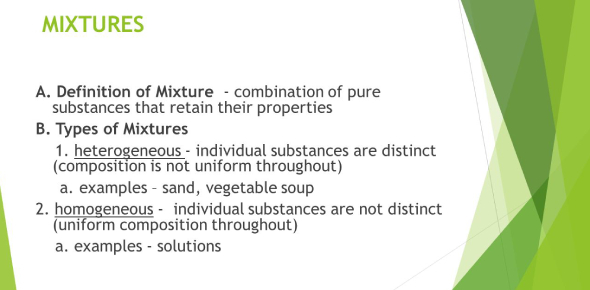# Chemistry Questions On Mixtures! 7th Grade Trivia Quiz

49 Questions | Total Attempts: 662SettingsMixtures can occur in different forms, and sometimes they create a solution that can be separated and other times the separation is not so easy. What have you learned so far about mixtures from your 7th-grade science class? Take up the test below and see if you need some more study hours or have all the knowledge on mixtures.

• 1.
A mixture is ...
• A.

A pure substancae

• B.

Two or more substances mixed together

• 2.
Which of the following are homogeneous mixtures?
• A.

Water

• B.

• C.

Shampoo

• D.

Oatmeal

• 3.
Which of the following are mechanical mixtures?
• A.

Chocolate bar with almonds

• B.

Chocolate milk

• C.

Flat pop

• D.

Orange juice with pulp

• 4.
What happens when you try to make a solution of salt and oil?
• A.

It becomes a homogeneous solution, the salt dissolves into the oil

• B.

It becomes a heterogeneous mixture, the salt doesn't dissovle into the oil

• C.

The oil can only dissolve a little bit of salt

• 5.
The formula for finding density is:
• A.

The volume divided by the mass.

• B.

The mass multiplied by the volume.

• C.

The mass divided by the volume.

• D.

The volume multiplied by the mass.

• 6.
You have two plastic vials. One contains 40ml of water and the other contains 40ml of alcohol. Which statement is correct?
• A.

Both vials have the same denisty.

• B.

Both vials have the same volume.

• C.

Both vials have the same mass.

• D.

All of the above.

• 7.
Mass is:
• A.

The amount of matter in an object.

• B.

The amount of matter in a small amount of space.

• C.

The amount of matter plus the volume.

• D.

The amount of matter that makes an object more dense.

• 8.
The density of an object is a______________
• A.

Chemical Property

• B.

Combustible Property

• C.

Physical Property

• D.

Reactive Property

• 9.
A pure substance always has ______ density when measured under _____ conditions.
• A.

The same; different

• B.

The same; the same

• C.

A different; the same

• D.

A different; different

• 10.
A solution is a type of_________
• A.

Matter

• B.

Mixture

• C.

Balance

• D.

Combustibility

• 11.
Which of the following does Not signal a chemical change?
• A.

Change in state

• B.

Production of gas

• C.

Color change

• D.

Production of light and heat

• 12.
Given a solution of sugar and water, and water and pepper, which is true.
• A.

If stired, the pepper would have the same solubility as sugar.

• B.

Pepper is soluble in water, bur sugar is not.

• C.

Sugar is soluble in water, but pepper is not.

• D.

Sugar and peper have the same solubility

• 13.
Other ____of substances, like color, size, and hardness, can be found without changing the substance into something else.
• A.

Reactivity

• B.

Physical properties

• C.

Evaporation

• D.

Chemical Properties

• 14.
A ____ has a definite volume but no definite shape.
• A.

Solid

• B.

Liquid

• C.

Gas

• D.

Matter

• E.

Density

• 15.
A ____ does not have a definite volume or a definite shape.
• A.

Gas

• B.

Solid

• C.

Liquid

• D.

Volume

• E.

Weight

• 16.
A _____ has both a definite volume and shape.
• A.

Liquid

• B.

Solid

• C.

Weight

• D.

Gas

• E.

Matter

• 17.
You can divide the mass of an object by its volume to find the object's ______.
• A.

Physical properties

• B.

Solubility

• C.

Reactivity

• D.

Density

• E.

Volume

• 18.
All material that takes up space is called.
• A.

Mass

• B.

Matter

• C.

Solubility

• D.

Weight

• E.

Volume

• 19.
The amount of material in an object is called the object's _____.
• A.

Physical properties

• B.

Density

• C.

Volume

• D.

Weight

• E.

Mass

• 20.
You can measure the amount of space a solid object takes up, or the _____ of a solid object, by placing the object in a graduated cylinder with water and measuring how much water is displaced.
• A.

Mass

• B.

Density

• C.

Volume

• D.

Weight

• E.

Solubility

• 21.
Sometimes the ability to be dissolved, or ________, can be used to identify a substance.
• A.

Solubility

• B.

Matter

• C.

Volume

• D.

Liquid

• E.

Gas

• 22.
PH is a measure of ____ ions.
• A.

Oxygen

• B.

Hydrogen

• C.

Hydroxide

• D.

None of the above

• 23.
Acids and bases can combine to create water and salts.
• A.

True

• B.

False

• 24.
Neutral solutions have a pH of
• A.

0

• B.

7

• C.

14

• D.

A neutral solution does not have a pH

• 25.
Which is NOT a property of a base?
• A.

Sour taste

• B.

Turns red litmus to blue

• C.

Slippery

• D.

Bitter taste

Related TopicsBack to top